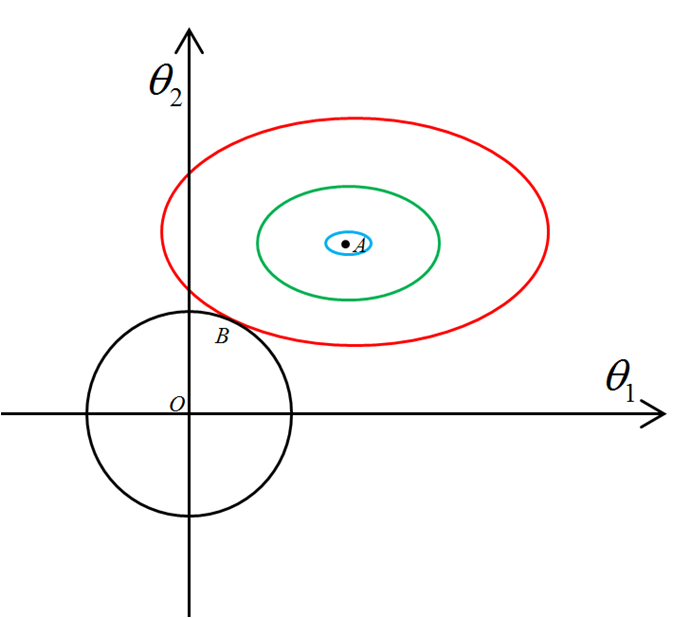# 理解深度学习中的正则化

1. 什么是正则化？
2. 为什么要正则化？
3. 怎样理解正则化？
4. 举例

$\begin{array}{}\text{(1.1)}& \stackrel{^}{J}\left(\omega ;X,y\right)=J\left(\omega ;X,y\right)+\frac{\alpha }{2}{\omega }^{T}\omega \end{array}$

$\begin{array}{}\text{(2.4)}& {\lambda }_{1}{\lambda }_{2}=1\end{array}$

$\ h_i(\theta) \$$\ g_j(\theta) \$写成向量的形式，$\ \vec{h}(\theta) \$$\ \vec{g}(\theta) \$，类比Lagrange乘子法，构造函数

$\begin{array}{}\text{(3.3)}& F\left(\theta ,\alpha ,\beta \right)=f\left(\theta \right)+\alpha \cdot \stackrel{\to }{h}\left(\theta \right)+\beta \cdot \stackrel{\to }{g}\left(\theta \right)\end{array}$

$\ \frac{\partial F}{\partial \theta}=0 \$的解$\ \theta(\alpha,\beta) \$ 代入式(3.3)，得到 $\ \tilde{F}(\alpha,\beta) \$，在一定约束下求其最大值，如式(3.4)所示，

$\begin{array}{}\text{(3.5)}& \alpha \cdot \stackrel{\to }{h}\left(\theta \right)=0\end{array}$

$\begin{array}{}\text{(3.6)}& \stackrel{~}{L}\left(\theta ,\alpha \right)=L\left(\theta \right)+\alpha \mathrm{\Omega }\left(\theta \right)\end{array}$

（上式可能有点难，可以自己推导一下）

$\begin{array}{}\text{(3.11)}& \stackrel{~}{\mathrm{\Omega }}\left(\theta \right)=\mathrm{\Omega }\left(\theta \right)-\mathrm{\Omega }\left(\theta \left(\alpha \right)\right)\end{array}$《深度学习》, Ian Goodfellow等, 人民邮电出版社；
《Numerical Recipes in C++》, William H.Press等, Cambridge University Press；